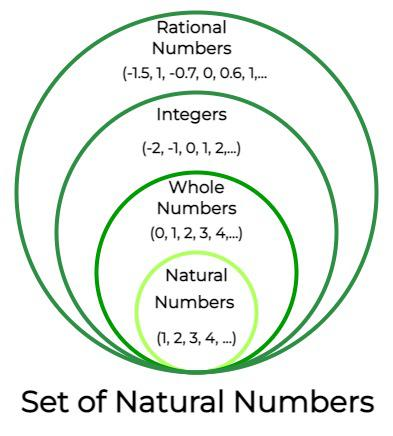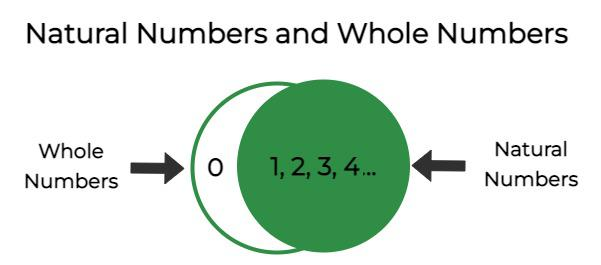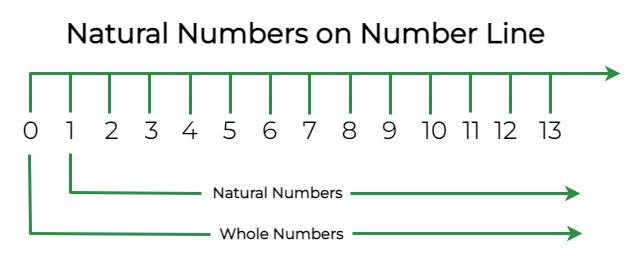GeeksforGeeks App
Open AppBrowser
Continue

## Related Articles

Natural numbers are all positive integers from 1 to infinity and are a component of the number system. Natural numbers are only positive integers, excluding zero, fractions, decimals, or negative numbers, and they are part of real numbers. Natural numbers are also called counting numbers. Let’s learn more about natural numbers, their properties, and examples.

## What are Natural Numbers?

Natural numbers or counting numbers are those integers that generate from 1 and go up to infinity. Numbers may be found everywhere, used for counting items, representing or trading money, calculating temperature, telling time, and so on. These numbers are referred to as “natural numbers” since they are used to count items. When counting items, it can be 5 glasses, 6 books, 1 bottle, and so on. Therefore, another name for natural numbers is counting numbers. A collection of all whole integers except 0 is referred to as natural numbers. These figures play an important role in daily actions and communication.

### Natural Numbers Definition

Natural numbers are those that can be counted and are a component of real numbers. Only positive integers, such as 1, 2, 3, 4, 5, 6, etc., are included in the set of natural numbers. Natural numbers start from 1 and go up to ∞.

### Set of Natural Numbers

In mathematics, the set of natural numbers is expressed as 1, 2, 3, … The set of natural numbers is represented by the symbol N. N = {1, 2, 3, 4, 5, … ∞}. One (1) is the lowest natural number. A collection of elements is referred to as a set (numbers in this context). The smallest element in N is 1, and the next element in terms of 1 and N for any element in N. 2 is 1 greater than 1, 3 is 1 greater than 2, and so on. The below table explains the different set forms of natural numbers.

Natural numbers are the subset of whole numbers, and whole numbers are the subset of integers. Similarly, integers are the subset of real numbers. The below-given diagram explains the relationship w.r.t. the sets of natural numbers, whole numbers, integers, and real numbers.### Examples of Natural Numbers

Non-negative integers are also known as natural numbers (all positive integers). 24, 57, 88, 979, 120502, and so forth are only a few instances. Will -4 be a Natural number? No. Since it is a negative integer. Will 3.6 be a natural number? No. Since it is not an Integer.

### Natural Even Numbers

Even natural numbers are those that are even, divisible by 2 precisely, and belong to the set N. So 2,4,6,8,… are examples of even natural numbers. The set of natural even numbers is represented as {2, 4, 6, 8, 10, 12, …}.

### Natural Odd Numbers

Natural numbers that are odd and belong to the set N are known as odd natural numbers, not divisible by 2 precisely. So 1, 3, 5, 7, … are examples of odd natural numbers. The set of natural odd numbers is represented as {1, 3, 5, 7, 9, 11, 13, …}.

### Smallest Natural Number

1 is known to be the smallest natural number. Natural numbers generate from 1 and terminate at ∞. While whole numbers generate from 0, therefore, the smallest whole number is 0.

### Natural Numbers from 1 to 100

The natural numbers are a part of whole numbers, natural number or counting numbers from 1 to 100 are 1, 2, 3, 4, 5, 6, 7, 8, 9, 10, 11, 12, 13, 14, 15, 16, 17, 18, 19, 20, 21, 22, 23, 24, 25, 26, 27, 28, 29, 30, 31, 32, 33, 34, 35, 36, 37, 38, 39, 40, 41, 42, 43, 44, 45, 46, 47, 48, 49, 50, 51, 52, 53, 54, 55, 56, 57, 58, 59, 60, 61, 62, 63, 64, 65, 66, 67, 68, 69, 70, 71, 72, 73, 74, 75, 76, 77, 78, 79, 80, 81, 82, 83, 84, 85, 86, 87, 88, 89, 90, 91, 92, 93, 94, 95, 96, 97, 98, 99, 100.

### Does 0 belong to Natural numbers?

The question is often asked if 0 is a natural number. Natural numbers are counting numbers. 0 is not a natural number. Since the counting begins at 1 instead of 0 when counting any number of items. The number 0 precisely belongs to the Whole number. 0 is also a part of Integers and is represented on the number line. However, even on the number line, everything from +1 and its right side belongs to Natural numbers.

## Natural Numbers and Whole Numbers

The set of whole numbers is identical to the set of natural numbers, with the exception that it includes a 0 as an extra number. In mathematics, the set of whole integers is expressed as 0, 1, 2, 3,… The letter W stands for it. It is clear from the definitions that any natural number is a whole number. Furthermore, all whole numbers other than 0 are natural numbers. Below are the representation of the sets of natural numbers and whole numbers in roaster form,

W = {0, 1, 2, 3, 4, 5, …}

N = {1, 2, 3, 4, 5, …}### Difference Between Natural Numbers and Whole Numbers

Natural numbers, such as 1, 2, 3, 4, and so on, are all positive numbers. They’re the numbers used for counting, and they go on indefinitely. The entire numbers, on the other hand, are all natural numbers, excluding zero, such as 1, 2, 3, 4, and so on. All whole numbers and their negative counterparts are considered integers. -4, -3, -2, -1, 0,1, 2, 3, 4, and so on are some examples. The table below explains the difference between natural numbers and whole numbers,

## Natural Numbers on a Number Line

On the number line, the set of natural numbers and whole numbers is shown below. Natural numbers are represented by all positive integers or integers on the right-hand side of 0, whereas whole numbers are represented by all positive integers plus zero. The below diagram shows natural numbers and whole numbers on the number line.### First 10 Natural Numbers

The first 10 natural numbers are 1, 2, 3, 4, 5, 6, 7, 8, 9, and 10. In the roaster set form, the first 10 natural numbers are represented as,

N = {1, 2, 3, 4, 5, 6, 7, 8, 9, 10}

## Properties of Natural Numbers

The properties of natural numbers have been derived from the properties of numbers. The four operations on natural numbers, addition, subtraction, multiplication, and division, result in four main characteristics of natural numbers, which are illustrated below:

• Closure property
• Commutative property
• Associative property
• Distributive property

### Closure Property

When two or more natural numbers are added and multiplied, the result is always a natural number. Addition Closure Property is a + b = c i.e. 3 + 2 = 5, 9 + 8 = 17. The sum of natural numbers is always a natural number, as this demonstrates. Multiplication Closure Property is ab = c i.e., 2 × 4 = 8, 7 × 8 = 56, etc. This demonstrates that a natural number is always the product of two natural numbers.

Note: Natural numbers may not obey the closure property when it comes to subtraction and division, which implies that subtracting or dividing two natural numbers may not result in a natural number.

### Associative Property

When adding and multiplying natural integers, the associative condition is true, i.e. a +(b + c) = (a + b) + c and a(b × c) = (a × b) c. Associative Property of Addition is a + (b + c) = (a + b) + c i.e; 1 + (3 + 5) = 1 + 8 = 9 and the same result is obtained in (1 + 3) + 5 = 4 + 5 = 9. Associative Property of Multiplication is a × (b × c) = (a × b) × c i.e; 2 × (2 × 1) = 2 × 2 = 4 and the same result is obtained in (a × b) × c = (2 × 2) × 1 = 4 × 1 = 4. Let’s brief both parts of the associative property of natural numbers,

• Associative property of addition: a + (b + c) = (a + b) + c
• Associative property of multiplication: a × (b × c) = (a × b) × c

Note: The associative property, on the other hand, does not hold true for natural number subtraction and division.

### Commutative Property

Even if the sequence of the numbers is changed, the sum or product of two natural numbers stays the same. The commutative property of N says that a + b = b + a and ab= ba for any a, b ∈ N. Let’s take a look at the commutative property of addition and commutative property of multiplication for natural numbers,

• Commutative Property of Addition: a + b = b + a ⇒ Example: 4 + 5 = 9 and b + a = 5 + 4 = 9.
• Commutative Property of Multiplication: a × b = b × a ⇒ Example: 3 × 2 = 6 and 2 × 3 = 6.

### Distributive Property

The distributive property for natural numbers has two types, distributive law of multiplication over addition and distributive law of multiplication over subtraction. If we are given a(b + c), then a can be distributed between b and c and becomes (ab + ac). Similarly, a(b – c) can become (ab – ac).

• Distributive law of multiplication over addition: a(b + c) = ab + ac.
• Distributive law of multiplication over multiplication: a(b – c) = ab – ac.

## Solved Examples on Natural Numbers

Example 1: Identify the natural numbers among the given numbers:

23, 98, 0, -98, 12.7, 11/7, 3.

Since negative numbers, 0, decimals, and fractions are not a part of natural numbers. Therefore, 0, -98, 12.7, and 11/7 are not natural numbers.

Therefore, natural numbers are 23, 98, and 3.

Example 2: Prove the distributive law of multiplication over addition with an example.

Distributive law of multiplication over addition states: a(b + c) = ab + ac.

For example, 4(10 + 20), here 4, 10, and 20 are all natural numbers and hence must follow the distributive law. Therefore,

4(10 + 20) = 4 × 10 + 4 × 20

4 × 30 = 40 + 80

120 = 120

Hence, proved.

Example 3: Prove the distributive law of multiplication over subtraction with an example.

Distributive law of multiplication over addition states: a(b – c) = ab – ac.

For example, 7(3 – 6), here 7, 3, and 6 are all natural numbers and hence must follow the distributive law. Therefore,

7(3 – 6) = 7 × 3 – 7 × 6

7 × -3 = 21 + 42

-21 = -21

Hence, proved.

Example 4: List the first 10 natural numbers.

1, 2, 3, 4, 5, 6, 7, 8, 9, and 10 are the first ten natural numbers.

Example 5: Identify the non-natural numbers among the given numbers:

45, 9, 10, -8, 1.17, 98/3, 33/11.

Since negative numbers, 0, decimals, and fractions are not a part of natural numbers. Therefore, -8, 1.17, 98/3 are non-natural numbers. Note that 33/11 can be simplified to 3 and 3 is a natural number.

Example 6: What is the variance of first 5 natural numbers?

Solution:

The formula for finding the variance of first n natural numbers is = (n2 – 1)/12

Putting n = 5,

= (52 -1)/12

= 24/12

= 2

## FAQs on Natural Numbers

Question 1: Every natural number is a whole number. True or False?

False. Every natural number is not a whole number as 0 is involved in whole numbers but not in natural numbers. Therefore, the assertion is wrong.

Question 2: Write down the sum of the first 10 natural numbers.

1, 2, 3, 4, 5, 6, 7, 8, 9, and 10 are the first ten natural numbers. Therefore, the sum of the first 10 natural numbers will be 1 + 2 + 3 + 4 + 5 + 6 + 7 + 8 + 9 + 10 = 55.

Question 3: Is 0 a natural number?

No, 0 is not a part of natural numbers. 0 is a part of whole numbers, and this is the major difference between whole numbers and natural numbers.

Question 4: What is the sum of first n natural numbers?

The formula for the sum of first n natural numbers is:

S = n (n + 1)/2

Here, n is the number of terms.

Question 5: What is the sum of squares of n natural numbers?

The formula for the sum of squares of n natural numbers is:

S = n(n + 1)(2n + 1)/6

Question 6: Which is the smallest natural number?

The smallest natural number is 1. Natural numbers begin at 1 and go up to infinity. Therefore, the smallest natural number is 1.

Question 7: Find the mean of first n natural numbers.